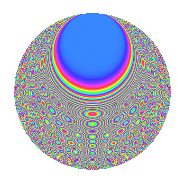# Properties

 Label 1350.2.uLevel 1350 Weight 2 Character orbit u Rep. character $$\chi_{1350}(49,\cdot)$$ Character field $$\Q(\zeta_{18})$$ Dimension 324 Sturm bound 540

# Related objects

## Defining parameters

 Level: $$N$$ = $$1350 = 2 \cdot 3^{3} \cdot 5^{2}$$ Weight: $$k$$ = $$2$$ Character orbit: $$[\chi]$$ = 1350.u (of order $$18$$ and degree $$6$$) Character conductor: $$\operatorname{cond}(\chi)$$ = $$135$$ Character field: $$\Q(\zeta_{18})$$ Sturm bound: $$540$$

## Dimensions

The following table gives the dimensions of various subspaces of $$M_{2}(1350, [\chi])$$.

Total New Old
Modular forms 1692 324 1368
Cusp forms 1548 324 1224
Eisenstein series 144 0 144

## Trace form

 $$324q + 12q^{6} - 24q^{9} + O(q^{10})$$ $$324q + 12q^{6} - 24q^{9} + 24q^{11} - 12q^{14} + 72q^{21} + 72q^{26} - 12q^{29} - 36q^{31} + 36q^{36} + 36q^{39} + 24q^{41} + 12q^{44} - 36q^{49} + 84q^{51} + 36q^{54} + 12q^{56} - 24q^{59} + 36q^{61} + 162q^{64} + 120q^{69} + 96q^{74} + 144q^{79} + 48q^{81} - 18q^{86} + 12q^{89} + 72q^{94} + 12q^{99} + O(q^{100})$$

## Decomposition of $$S_{2}^{\mathrm{new}}(1350, [\chi])$$ into newform subspaces

The newforms in this space have not yet been added to the LMFDB.

## Decomposition of $$S_{2}^{\mathrm{old}}(1350, [\chi])$$ into lower level spaces

$$S_{2}^{\mathrm{old}}(1350, [\chi]) \cong$$ $$S_{2}^{\mathrm{new}}(135, [\chi])$$$$^{\oplus 4}$$$$\oplus$$$$S_{2}^{\mathrm{new}}(270, [\chi])$$$$^{\oplus 2}$$$$\oplus$$$$S_{2}^{\mathrm{new}}(675, [\chi])$$$$^{\oplus 2}$$

## Hecke Characteristic Polynomials

There are no characteristic polynomials of Hecke operators in the database